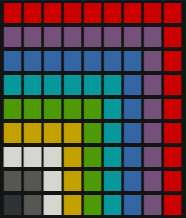# pixelpuzzle

Puzzle game for the R console. Restore the pixel art by shifting rows!## Installation

CRAN

``install.packages("pixelpuzzle")``

Github

``````# install from github
if (!require(devtools)) install.packages("devtools")
devtools::install_github("rolkra/pixelpuzzle")``````

# Start Game

``````library(pixelpuzzle)
pixelpuzzle()``````

# Pixel Art

{pixelpuzzle} comes with predefined pixel art you can play with. Just start the game with `pixelpuzzle()` to select one.

In case you want to define your own pixel art you can use the following color codes for each pixel:

• R = Red
• M = Magenta
• B = Blue
• C = Cyan
• G = Green
• Y = Yellow
• W = White
• S = Silver
• X = Black
• . = No color (transparent)
``````library(pixelpuzzle)

sprite <- c(
"RRRRRRRRR",
"MMMMMMMMR",
"BBBBBBBMR",
"CCCCCCBMR",
"GGGGGCBMR",
"YYYYGCBMR",
"WWWYGCBMR",
"SSWYGCBMR",
"XSWYGCBMR"
)

show_sprite(sprite)``````You can use this pixel art by passing it as parameter to `pixelpuzzle()`

``pixelpuzzle(sprite)``